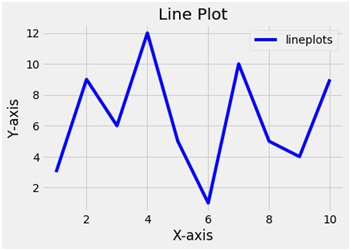# Python | Create a line plot using matplotlib.pyplot

Here, we are implementing a python program to create a line plot using matplotlib.pyplot.
Submitted by Ayush Sharma, on November 14, 2018

Problem statement: Write a program in python (using matplotlib.pyplot) to create a line plot.

Program:

```import matplotlib.pyplot as plt

x = [1,2,3,4,5,6,7,8,9,10]
y = [3,9,6,12,5,1,10,5,4,9]
plt.plot(x,y, label='lineplots', color='b')
plt.xlabel('X-axis')
plt.ylabel('Y-axis')
plt.title('Line Plot')
plt.legend()
plt.show()
```

OutputExplanation:

Python library matplotlib.pyplot is used to draw the above chart. Two random variables x and y are taken with random values. The plot function plots a line plot. The plot function takes 2 arguments i.e. x and y and a label variable gives the label to the plot. To name the axes xlabel and ylabel functions are used and to give the title to the plot the title function is used. To show the legend the legend function is used and finally to show the plot the show functions.

Preparation